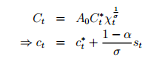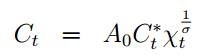# How does international risk sharing affects the DSGE model results?

In SOE models, there is international risk sharing in the international market. A common form is shown below where consumption depends on the consumption in the rest of the world and the real exchange rate.However, this equation does not appear in the model’s solution (written in DYNARE). Why is there the need to mention it in the paper as it does not appear to be used in DYNARE?

Let me ask though, what risk is being shared here, intuitively. I know in some macro models (not DSGE), households share risk so that individual consumption varies only with aggregate income (not idiosyncratic income), helping to mitigate the effect of wide volatilities in one’s income on his/her consumption. So in the equations above, I guess if consumption varies with c^*_t and s_t, the household is mitigating some kind of risk. But intuitively I cannot see what risk it is.

2 Likes
1. Which equation is not put into the models?
2. You are sharing risks across countries. The logic is the same as between individuals. Here, countries are subject to country-specific shocks. Part of the risk can be diversified by a risk-sharing arrangement that equalizes marginal utilities across countries.
1 Like

Oh, I see. Many thanks!! I could not see c^*_t in DYNARE (for the paper I read), so I thought c_t = c^*_t + \frac{1-\alpha}{\sigma} s_t was not used. But I see my mistake, i.e., the global goods market clears (C^*_t =Y^*_t), and international risk sharing is being captured through the goods market-clearing condition: Y_t(i) = C^h_t(i) + X_t(i), where X_t = v(\frac{P^h_t}{S_t P^*_t})^{-\eta}C^*_t.

Wanted a hint though how international risk sharing assumption affects the DSGE model results because I see some SOE models with no international risk sharing like this one (NTG.pdf (243.8 KB)).

My current model has no international risk sharing, If I assume there is international risk sharing, I guess I can do something like this?
NX_t = X_t - Imports = v(\frac{P^h_t}{S_t P^*_t})^{-\eta}C^*_t - Imports
And then I can replace C^*_t using the risk sharing condition:I am not writing the NX equation in full though. But hopefully, it isn’t confusing. Thanks!!

Sorry for bothering your discussion. I remember the international risk sharing condition is associated with the complete international financial market assumption (Arrow securities), while in your attachment, the budget constraint is an incomplete type.

1 Like

Oh nice! Many thanks! I think my model is ok then (i.e., without international risk sharing) since the foreign asset in the household’s budget constraint (in my model) is not Arrow securities.

May I ask though, how does it affect the DSGE model results whether or not assets are assumed to be Arrow securities? Maybe arrow securities are more realistic, but any other known benefits regarding the simulation of the model?

@HelloDynare Risk sharing can be complete or partial. Full risk sharing indeed involves Arrow or Arrow-Debreu securities. It implies that marginal utilities are equalized across all states of the world.
Incomplete risk sharing via non-contingent securities only allows for limited risk-sharing. There will be some equalization of marginal utilities but it will be incomplete, i.e. not be state by state, but rather on average (the Euler equation features an expectation on the right)
@kofiemma Complete international financial markets are definitely unrealistic. Incomplete markets usually perform better. See e.g.

2 Likes